# Sort rows or columns in Pandas Dataframe based on values

In this article, Let’s discuss how to Sort rows or columns in Pandas Dataframe based on values. Pandas `sort_values() `method sorts a data frame in Ascending or Descending order of passed Column. It’s different than the sorted Python function since it cannot sort a data frame and particular column cannot be selected.

Syntax: DataFrame.sort_values(by, axis=0, ascending=True, inplace=False, kind=’quicksort’, na_position=’last’)

Parameters: This method will take following parameters :
by: Single/List of column names to sort Data Frame by.
axis: 0 or ‘index’ for rows and 1 or ‘columns’ for Column.
ascending: Boolean value which sorts Data frame in ascending order if True.
inplace: Boolean value. Makes the changes in passed data frame itself if True.
kind: String which can have three inputs(‘quicksort’, ‘mergesort’ or ‘heapsort’) of the algorithm used to sort data frame.
na_position: Takes two string input ‘last’ or ‘first’ to set position of Null values. Default is ‘last’.

Return Type: Returns a sorted Data Frame with Same dimensions as of the function caller Data Frame.

Now, Let’s create a sample dataframe :

 `# import pandas library as pd ` `import` `pandas as pd ` ` `  `# List of Tuples ` `students ``=` `[(``'Ankit'``, ``22``, ``'Up'``, ``'Geu'``), ` `           ``(``'Ankita'``, ``31``, ``'Delhi'``, ``'Gehu'``), ` `           ``(``'Rahul'``, ``16``, ``'Tokyo'``, ``'Abes'``), ` `           ``(``'Simran'``, ``41``, ``'Delhi'``, ``'Gehu'``), ` `           ``(``'Shaurya'``, ``33``, ``'Delhi'``, ``'Geu'``), ` `           ``(``'Harshita'``, ``35``, ``'Mumbai'``, ``'Bhu'` `), ` `           ``(``'Swapnil'``, ``35``, ``'Mp'``, ``'Geu'``), ` `           ``(``'Priya'``, ``35``, ``'Uk'``, ``'Geu'``), ` `           ``(``'Jeet'``, ``35``, ``'Guj'``, ``'Gehu'``), ` `           ``(``'Ananya'``, ``35``, ``'Up'``, ``'Bhu'``) ` `            ``] ` ` `  `# Create a DataFrame object from ` `# list of tuples with columns ` `# and indices. ` `details ``=` `pd.DataFrame(students, columns ``=``[``'Name'``, ``'Age'``,  ` `                                           ``'Place'``, ``'College'``], ` `                        ``index ``=``[ ``'b'``, ``'c'``, ``'a'``, ``'e'``, ``'f'``, ` `                                ``'g'``, ``'i'``, ``'j'``, ``'k'``, ``'d'``]) ` `# show the dataframe ` `details `

Output: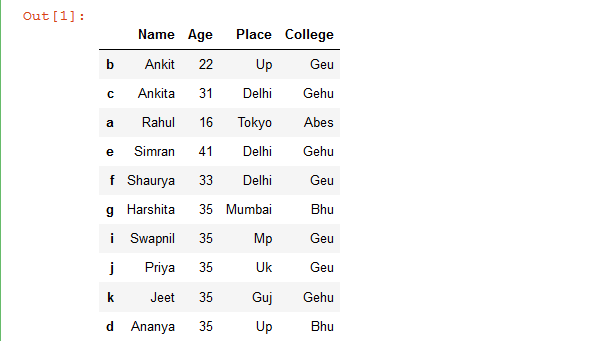Example 1: Sort Dataframe rows based on a single column.

 `# import pandas library as pd ` `import` `pandas as pd ` ` `  `# List of Tuples ` `students ``=` `[(``'Ankit'``, ``22``, ``'Up'``, ``'Geu'``), ` `           ``(``'Ankita'``, ``31``, ``'Delhi'``, ``'Gehu'``), ` `           ``(``'Rahul'``, ``16``, ``'Tokyo'``, ``'Abes'``), ` `           ``(``'Simran'``, ``41``, ``'Delhi'``, ``'Gehu'``), ` `           ``(``'Shaurya'``, ``33``, ``'Delhi'``, ``'Geu'``), ` `           ``(``'Harshita'``, ``35``, ``'Mumbai'``, ``'Bhu'` `), ` `           ``(``'Swapnil'``, ``35``, ``'Mp'``, ``'Geu'``), ` `           ``(``'Priya'``, ``35``, ``'Uk'``, ``'Geu'``), ` `           ``(``'Jeet'``, ``35``, ``'Guj'``, ``'Gehu'``), ` `           ``(``'Ananya'``, ``35``, ``'Up'``, ``'Bhu'``) ` `            ``] ` ` `  `# Create a DataFrame object from ` `# list of tuples with columns ` `# and indices. ` `details ``=` `pd.DataFrame(students, columns ``=``[``'Name'``, ``'Age'``, ` `                                           ``'Place'``, ``'College'``], ` `                        ``index ``=``[ ``'b'``, ``'c'``, ``'a'``, ``'e'``, ``'f'``, ` `                                ``'g'``, ``'i'``, ``'j'``, ``'k'``, ``'d'``]) ` ` `  `# Sort the rows of dataframe by 'Name' column ` `rslt_df ``=` `details.sort_values(by ``=` `'Name'``) ` ` `  `# show the resultant Dataframe ` `rslt_df `

Output: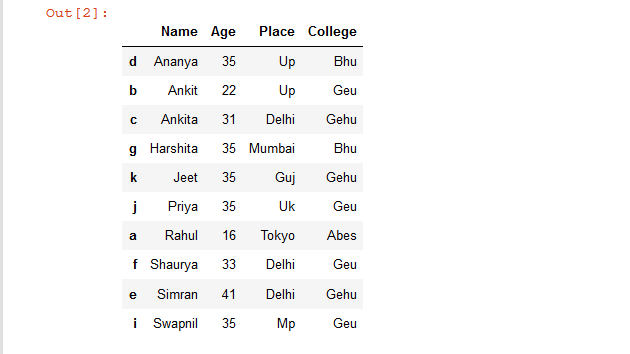Example 2: Sort Dataframe rows based on a multiple columns.

 `# import pandas library as pd ` `import` `pandas as pd ` ` `  `# List of Tuples ` `students ``=` `[(``'Ankit'``, ``22``, ``'Up'``, ``'Geu'``), ` `           ``(``'Ananya'``, ``31``, ``'Delhi'``, ``'Gehu'``), ` `           ``(``'Rahul'``, ``16``, ``'Tokyo'``, ``'Abes'``), ` `           ``(``'Simran'``, ``41``, ``'Delhi'``, ``'Gehu'``), ` `           ``(``'Shaurya'``, ``33``, ``'Delhi'``, ``'Geu'``), ` `           ``(``'Harshita'``, ``35``, ``'Mumbai'``, ``'Bhu'` `), ` `           ``(``'Priya'``, ``35``, ``'Mp'``, ``'Geu'``), ` `           ``(``'Priya'``, ``34``, ``'Uk'``, ``'Geu'``), ` `           ``(``'Jeet'``, ``35``, ``'Guj'``, ``'Gehu'``), ` `           ``(``'Ananya'``, ``35``, ``'Up'``, ``'Bhu'``) ` `            ``] ` ` `  `# Create a DataFrame object from ` `# list of tuples with columns ` `# and indices. ` `details ``=` `pd.DataFrame(students, columns ``=``[``'Name'``, ``'Age'``,  ` `                                           ``'Place'``, ``'College'``], ` `                        ``index ``=``[ ``'b'``, ``'c'``, ``'a'``, ``'e'``, ``'f'``, ` `                                ``'g'``, ``'i'``, ``'j'``, ``'k'``, ``'d'``]) ` ` `  `# sort Dataframe rows based on a 'Name' & 'Age' columns ` ` `  `# if duplicate value is present in 'Name' column ` `# then sorting will be done according to 'Age' column ` `rslt_df ``=` `details.sort_values(by ``=` `[``'Name'``, ``'Age'``]) ` ` `  `# show the resultant Dataframe ` `rslt_df `

Output: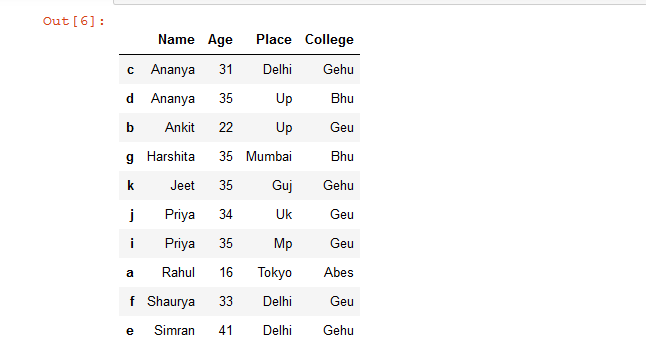Example 3: Sort Dataframe rows based on columns in Descending Order.

 `# import pandas library as pd ` `import` `pandas as pd ` ` `  `# List of Tuples ` `students ``=` `[(``'Ankit'``, ``22``, ``'Up'``, ``'Geu'``), ` `           ``(``'Ananya'``, ``31``, ``'Delhi'``, ``'Gehu'``), ` `           ``(``'Rahul'``, ``16``, ``'Tokyo'``, ``'Abes'``), ` `           ``(``'Simran'``, ``41``, ``'Delhi'``, ``'Gehu'``), ` `           ``(``'Shaurya'``, ``33``, ``'Delhi'``, ``'Geu'``), ` `           ``(``'Harshita'``, ``35``, ``'Mumbai'``, ``'Bhu'` `), ` `           ``(``'Priya'``, ``35``, ``'Mp'``, ``'Geu'``), ` `           ``(``'Priya'``, ``34``, ``'Uk'``, ``'Geu'``), ` `           ``(``'Jeet'``, ``35``, ``'Guj'``, ``'Gehu'``), ` `           ``(``'Ananya'``, ``35``, ``'Up'``, ``'Bhu'``) ` `            ``] ` ` `  `# Create a DataFrame object from ` `# list of tuples with columns ` `# and indices. ` `details ``=` `pd.DataFrame(students, columns ``=``[``'Name'``, ``'Age'``, ` `                                           ``'Place'``, ``'College'``], ` `                        ``index ``=``[ ``'b'``, ``'c'``, ``'a'``, ``'e'``, ``'f'``,  ` `                                ``'g'``, ``'i'``, ``'j'``, ``'k'``, ``'d'``]) ` ` `  `# sort Dataframe rows based on "Name'  ` `# column in Descending Order ` `rslt_df ``=` `details.sort_values(by ``=` `'Name'``, ascending ``=` `False``) ` ` `  `# show the resultant Dataframe ` `rslt_df `

Output: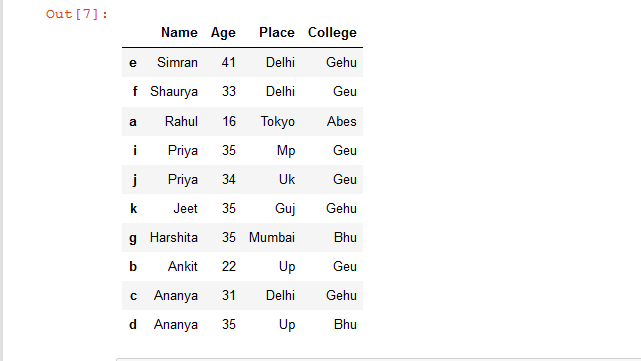Example 4: Sort Dataframe rows based on a column in Place.

 `# import pandas library as pd ` `import` `pandas as pd ` ` `  `# List of Tuples ` `students ``=` `[(``'Ankit'``, ``22``, ``'Up'``, ``'Geu'``), ` `           ``(``'Ananya'``, ``31``, ``'Delhi'``, ``'Gehu'``), ` `           ``(``'Rahul'``, ``16``, ``'Tokyo'``, ``'Abes'``), ` `           ``(``'Simran'``, ``41``, ``'Delhi'``, ``'Gehu'``), ` `           ``(``'Shaurya'``, ``33``, ``'Delhi'``, ``'Geu'``), ` `           ``(``'Harshita'``, ``35``, ``'Mumbai'``, ``'Bhu'` `), ` `           ``(``'Priya'``, ``35``, ``'Mp'``, ``'Geu'``), ` `           ``(``'Priya'``, ``34``, ``'Uk'``, ``'Geu'``), ` `           ``(``'Jeet'``, ``35``, ``'Guj'``, ``'Gehu'``), ` `           ``(``'Ananya'``, ``35``, ``'Up'``, ``'Bhu'``) ` `            ``] ` ` `  `# Create a DataFrame object from ` `# list of tuples with columns ` `# and indices. ` `details ``=` `pd.DataFrame(students, columns ``=``[``'Name'``, ``'Age'``, ` `                                           ``'Place'``, ``'College'``], ` `                        ``index ``=``[ ``'b'``, ``'c'``, ``'a'``, ``'e'``, ``'f'``, ` `                                ``'g'``, ``'i'``, ``'j'``, ``'k'``, ``'d'``]) ` ` `  `# Sort the rows of dataframe by  'Name'  ` `# column inplace ` `details.sort_values(by ``=` `'Name'``, inplace ``=` `True``) ` ` `  `# show the resultant Dataframe ` `details `

Output: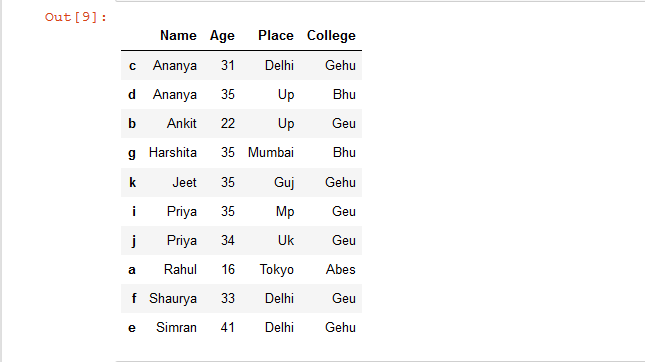Let’s see another simple Dataframe on which we are able to sort columns based on rows.

 `# import pandas library as pd ` `import` `pandas as pd ` ` `  `# List of Tuples ` `students ``=` `[ ` `           ``(``75``, ``50``, ``60``, ``70``), ` `           ``(``75``, ``55``, ``65``, ``75``), ` `           ``(``75``, ``35``, ``45``, ``25``), ` `           ``(``75``, ``90``, ``60``, ``70``), ` `           ``(``76``, ``90``, ``70``, ``60``), ` `           ``(``90``, ``80``, ``70``, ``60``), ` `           ``(``65``, ``10``, ``30``, ``20``) ` `            ``] ` ` `  `# Create a DataFrame object from ` `# list of tuples with columns ` `# and indices. ` `details ``=` `pd.DataFrame(students, columns ``=``[``'Hindi'``, ``'Math'``,  ` `                                           ``'Science'``, ``'English'``], ` `                        ``index ``=` `[``'Ankit'``, ``'Rahul'``, ``'Aishwarya'``,  ` `                                 ``'Shivangi'``, ``'Priya'``, ``'Swapnil'``, ` `                                 ``'Shaurya'``]) ` `# show the dataframe ` `details `

Output: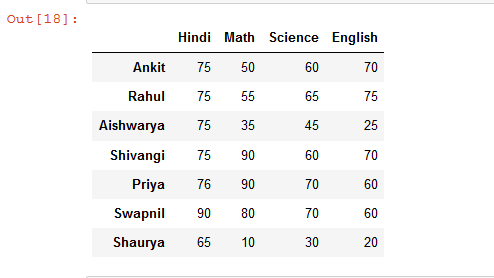Example 1: Sort columns of a Dataframe based on a single row.

 `# import pandas library as pd ` `import` `pandas as pd ` ` `  `# List of Tuples ` `students ``=` `[ ` `           ``(``75``, ``50``, ``60``, ``70``), ` `           ``(``75``, ``55``, ``65``, ``75``), ` `           ``(``75``, ``35``, ``45``, ``25``), ` `           ``(``75``, ``90``, ``60``, ``70``), ` `           ``(``76``, ``90``, ``70``, ``60``), ` `           ``(``90``, ``80``, ``70``, ``60``), ` `           ``(``65``, ``10``, ``30``, ``20``) ` `            ``] ` ` `  `# Create a DataFrame object from ` `# list of tuples with columns ` `# and indices. ` `details ``=` `pd.DataFrame(students, columns ``=``[``'Hindi'``, ``'Math'``,  ` `                                           ``'Science'``, ``'English'``], ` `                        ``index ``=` `[``'Ankit'``, ``'Rahul'``, ``'Aishwarya'``,  ` `                                 ``'Shivangi'``, ``'Priya'``, ``'Swapnil'``, ` `                                 ``'Shaurya'``]) ` ` `  `# sort columns of a Dataframe based  ` `# on a 'Shivangi' row ` `rslt_df ``=` `details.sort_values(by ``=` `'Shivangi'``, axis ``=` `1``) ` ` `  `# show the dataframe ` `rslt_df `

Output: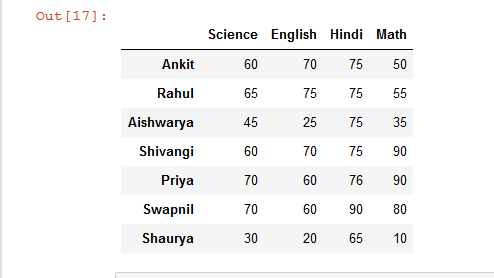Example 2: Sort columns of a Dataframe in Descending Order based on a single row.

 `# import pandas library as pd ` `import` `pandas as pd ` ` `  `# List of Tuples ` `students ``=` `[ ` `           ``(``75``, ``50``, ``60``, ``70``), ` `           ``(``75``, ``55``, ``65``, ``75``), ` `           ``(``75``, ``35``, ``45``, ``25``), ` `           ``(``75``, ``90``, ``60``, ``70``), ` `           ``(``76``, ``90``, ``70``, ``60``), ` `           ``(``90``, ``80``, ``70``, ``60``), ` `           ``(``65``, ``10``, ``30``, ``20``) ` `            ``] ` ` `  `# Create a DataFrame object from ` `# list of tuples with columns ` `# and indices. ` `details ``=` `pd.DataFrame(students, columns ``=``[``'Hindi'``, ``'Math'``,  ` `                                           ``'Science'``, ``'English'``], ` `                        ``index ``=` `[``'Ankit'``, ``'Rahul'``, ``'Aishwarya'``,  ` `                                 ``'Shivangi'``, ``'Priya'``, ``'Swapnil'``, ` `                                 ``'Shaurya'``]) ` ` `  `# Sort columns of a dataframe in descending order  ` `# based on a 'Shivangi' row  ` `rslt_df ``=` `details.sort_values(by ``=` `'Shivangi'``, axis ``=` `1``, ascending ``=` `False``) ` ` `  `rslt_df `

Output: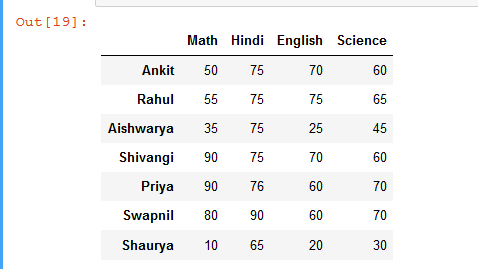Example 3: Sort columns of a Dataframe based on a multiple rows.

 `# import pandas library as pd ` `import` `pandas as pd ` ` `  `# List of Tuples ` `students ``=` `[ ` `           ``(``75``, ``50``, ``60``, ``70``), ` `           ``(``75``, ``55``, ``65``, ``75``), ` `           ``(``75``, ``35``, ``45``, ``25``), ` `           ``(``75``, ``90``, ``60``, ``70``), ` `           ``(``76``, ``90``, ``70``, ``60``), ` `           ``(``90``, ``80``, ``70``, ``60``), ` `           ``(``65``, ``10``, ``30``, ``20``) ` `            ``] ` ` `  `# Create a DataFrame object from ` `# list of tuples with columns ` `# and indices. ` `details ``=` `pd.DataFrame(students, columns ``=``[``'Hindi'``, ``'Math'``,  ` `                                           ``'Science'``, ``'English'``], ` `                        ``index ``=` `[``'Ankit'``, ``'Rahul'``, ``'Aishwarya'``,  ` `                                 ``'Shivangi'``, ``'Priya'``, ``'Swapnil'``, ` `                                 ``'Shaurya'``]) ` ` `  `# sort Dataframe columns based on a 'Shivangi' & 'Priya' rows ` ` `  `# if duplicate value is present in 'Shivangi'  row ` `# then sorting will be done according to 'Priya' row ` `rslt_df ``=` `details.sort_values(by ``=` `[``'Shivangi'``, ``'Priya'``], axis ``=` `1``) ` ` `  `rslt_df `

Output: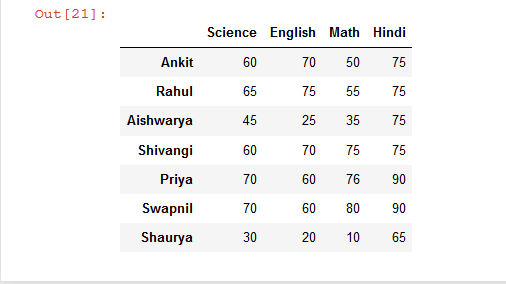My Personal Notes arrow_drop_upCheck out this Author's contributed articles.

If you like GeeksforGeeks and would like to contribute, you can also write an article using contribute.geeksforgeeks.org or mail your article to contribute@geeksforgeeks.org. See your article appearing on the GeeksforGeeks main page and help other Geeks.

Please Improve this article if you find anything incorrect by clicking on the "Improve Article" button below.

Article Tags :

Be the First to upvote.

Please write to us at contribute@geeksforgeeks.org to report any issue with the above content.